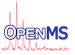OpenMS  2.8.0
NonNegativeLeastSquaresSolver Class Reference

Wrapper for a non-negative least squares (NNLS) solver. More...

`#include <OpenMS/MATH/MISC/NonNegativeLeastSquaresSolver.h>`

## Public Types

enum  RETURN_STATUS { SOLVED , ITERATION_EXCEEDED }

## Static Public Member Functions

static Int solve (const Matrix< double > &A, const Matrix< double > &b, Matrix< double > &x)
This is a wrapper for the external nnls library for the non-negative least square problem Ax=b, where x>0. More...

## Detailed Description

Wrapper for a non-negative least squares (NNLS) solver.

It solves Ax=b, where x>0 in the least squares sense (i.e. minimum residual)

## ◆ RETURN_STATUS

 enum RETURN_STATUS
Enumerator
SOLVED
ITERATION_EXCEEDED

## ◆ solve()

 static Int solve ( const Matrix< double > & A, const Matrix< double > & b, Matrix< double > & x )
static

This is a wrapper for the external nnls library for the non-negative least square problem Ax=b, where x>0.

Parameters
 A Input matrix A of size mxn b Input vector (matrix with one column) b of size mx1 x Output vector with non-negative least square solution of size mx1
Returns
status of solution (either NonNegativeLeastSquaresSolver::SOLVED, NonNegativeLeastSquaresSolver::ITERATION_EXCEEDED)
Exceptions
 Exception::InvalidParameters if Matrix dimensions do not fit